Discrete Dynamics Lab

# Multi-Value DDLab

#### Some examples, click to enlarge DDLab home DD-Life Self-reproduction update summary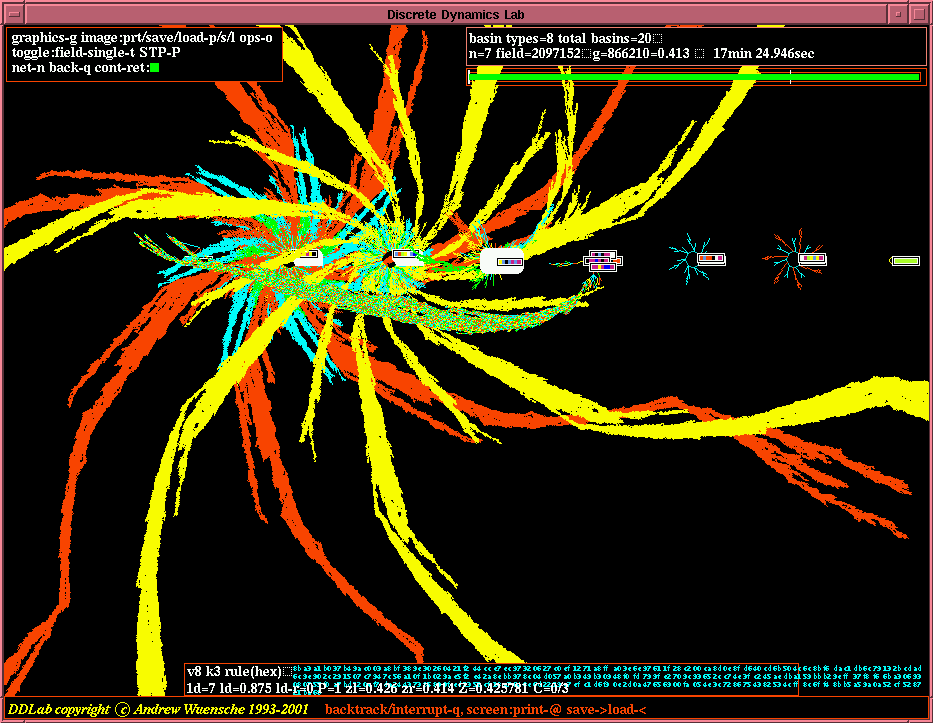CA basin of attraction field, n=7, k=3 with a value range v=8 (color-keys below for black and white backgrounds). The look-up table was chosen at random. with a Z-parameter=0.424781. Just the 8 non-equivalent basins are shown from a total of 20, and the non-equivalent attractor states. State-space = 7^8 = 2097152.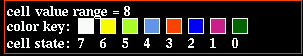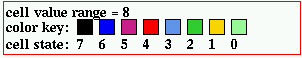The multi-value version of DDLab allows network elements to have a value range v anywhere from 2 to 8. All DDLab functions (as described in the manual) have been generalised for multi-value, including setting rules and initial states, attractor basins including compression, space-time patterns, filtering, frozen cells, graph layout, density plots (each density is shown), look_up histogram, input-entropy, entropy-density plots, automatic classification and samples of glider rules (include in dd_extra.zip), the reverse algorithms for CA, RBN and random maps, the Z and lambda parameters, learning algorithms, the meta-graph, canalising, the Derrida plot, and damage spread. Some new functions include: Post functions, symmetric and k-totalistic rules for 1d, 2d and 3d, "un-slanting" 1d space-time patterns, and hexagonal neighborhoods/lattice. Click here for a summary of changes. Click here for examples and details of DD-Life, families of "Life"-like and other complex 2d and 3d CA rules Click on the images below to see them full size.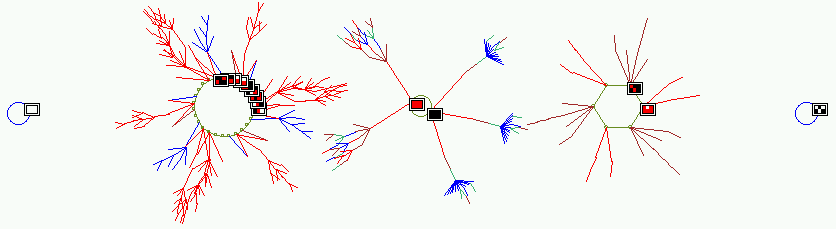The basin of attraction field of a n=6, k=3 CA with a value range v=3 (i.e. values 0,1,2 colored white, red and black). The look-up table is 222211010010021221122111221 with a Z-parameter=0.37037. Just the 5 non-equivalent basins are shown from a total of 8, and non-equivalent attractor states are shown as 2d 3x2 patterns. State-space = 3^6 = 729.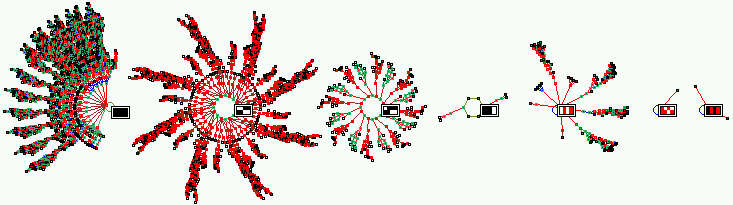The basin of attraction field of a n=8, k=3 CA with a value range v=3 (i.e. values 0,1,2 colored white, red and black). The look-up table is 020120102021201201011011022 with a Z-parameter=0.7037. Just the 7 non-equivalent basins are shown from a total of 17, and one attractor state in each basin is shown as 2d 4x2 pattern. State-space = 3^8 = 6561.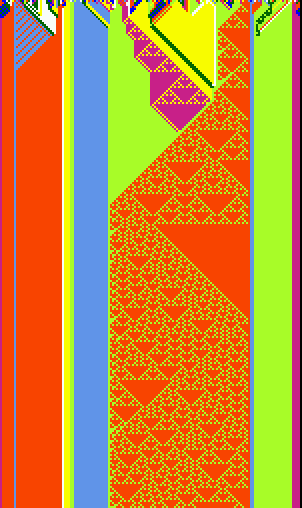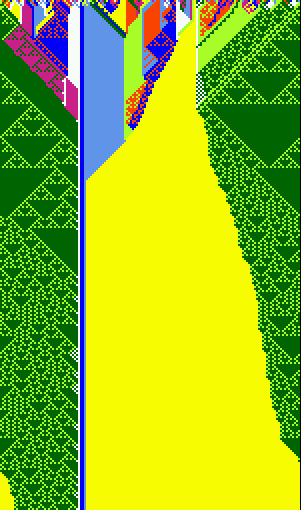1d space-time patterns from random initial states. n=150, v=8, k=4, 1d CA, showing the evolution of a generalised Altenberg rule, where the probability of a look-up table output is equal to the frequency of values in its neighborhood.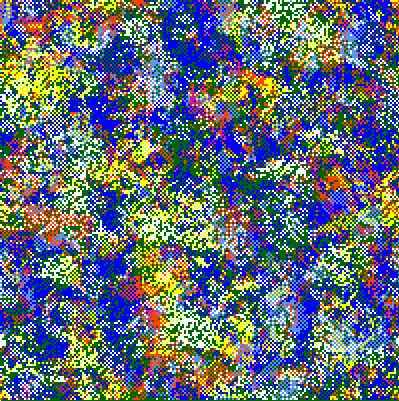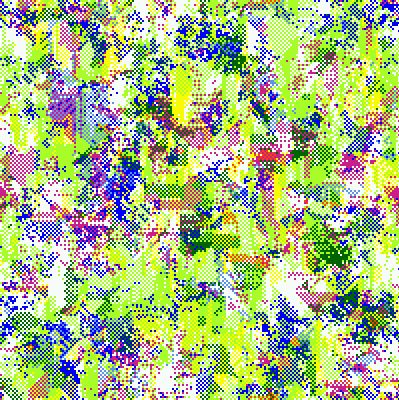Evolved 2d patterns from random initial states. n=200x200, v=8, k=4, 2d CA, for two generalised Altenberg rules, where the probability of a look-up table output is equal to the frequency of values in its neighborhood.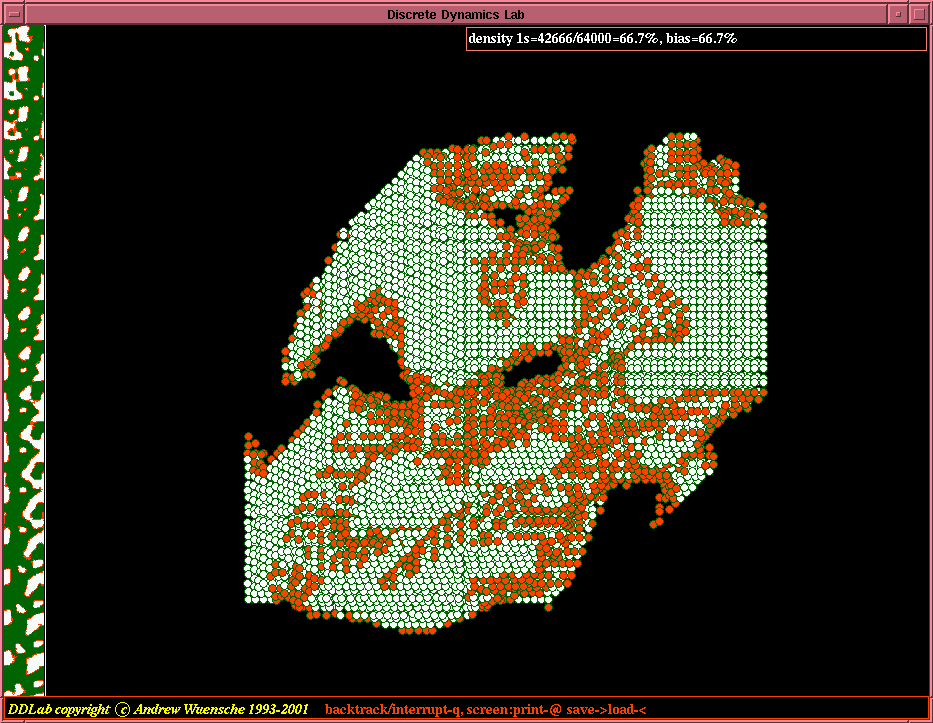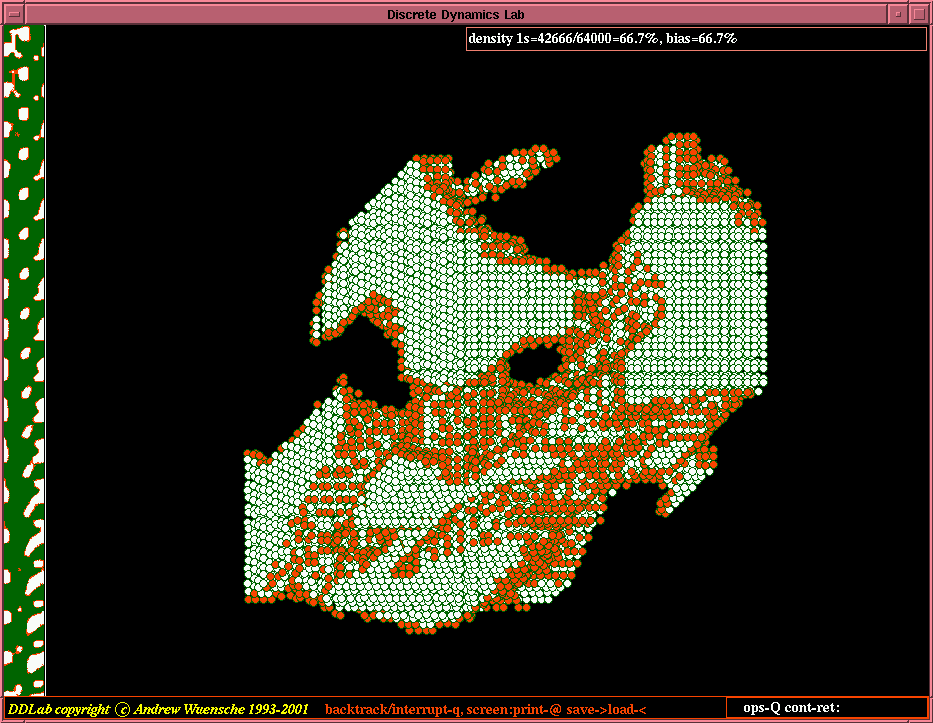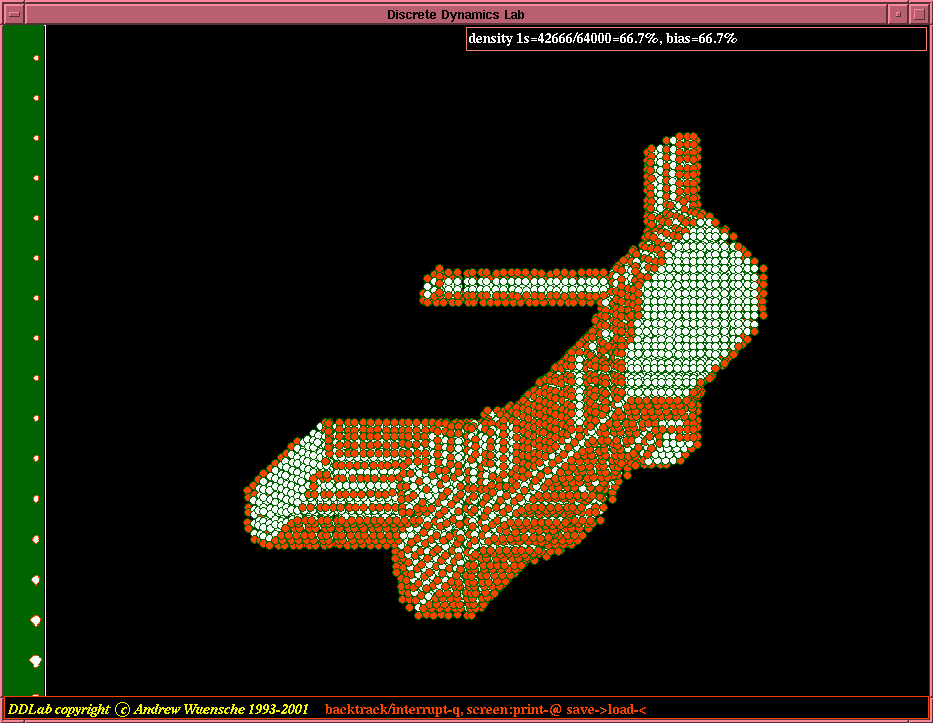A 3d, 40x40x40, v=3, k=7, CA, values 0,1,2 colored white, red and black, showing the evolution according to the totalistic rule, code 2222122111101100000. Horizontal x-sections of the 3d network are shown on the left.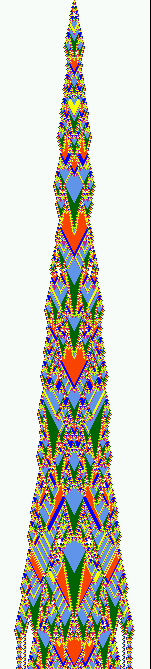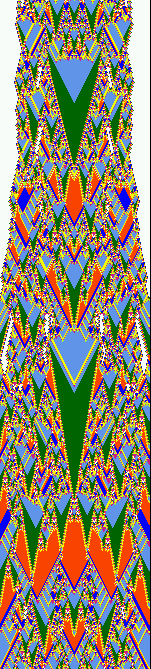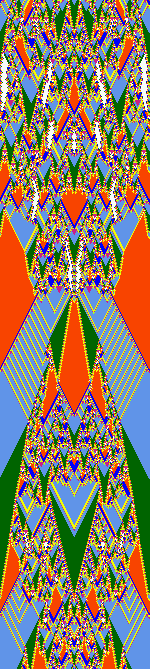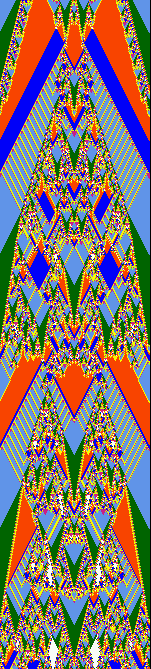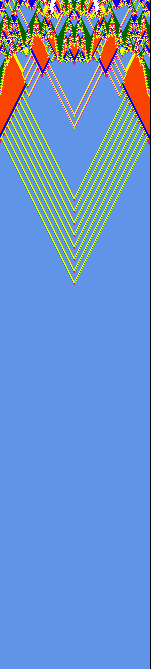An example of the space-time patterns of a 1d symmetric rule, v=8, k=2, n=150. The seed is a single 6 (yellow) in a uniform background of 7s (white). There are 670 time-steps in each panel, which follow on from each other. In the 5th panel the dynamics reaches the all 4s (light blue) point attractor. This is a "symmetric/legal" rule, where "symmetric" means that reflected neighborhoods have the same output in the lookup table, and "legal" (after Wolfram) means a symmetric rule where the uniform neighborhoods output their corresponding color (i.e. 000->0, 111->1, 222-2 etc). Setting such rules at random is a new feature in multi-value DDLab. The example also illustrate another new feature, the ability to "un-slant" k=2 (or any even k) space-time patterns by rotating successive rows by 1/2 cell width. This maintains the appearance of symmetry for even k and makes a k2 system equivalent to a triangular lattice.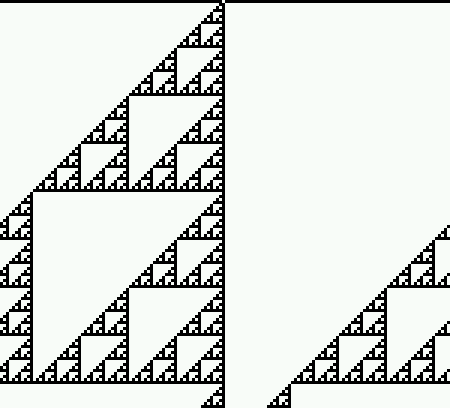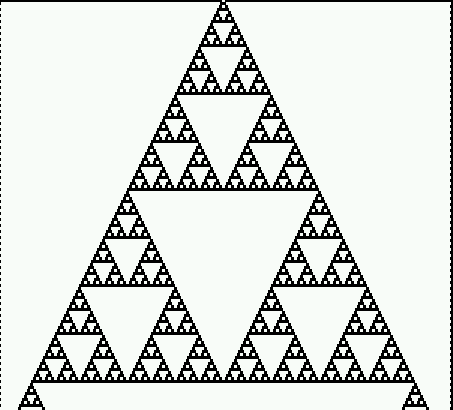The "un-slant" option can be applied irrespective of k. Odd k rules are sometime effectively even k rules, such as the v2k3 cluster of equivalent rules 60,195,102,153 (the equivalent v2k2 cluster is 6,9). On the left is rule 153 as normally seen, on the right "un-slanted". n=150, 197 time-steps from seed of a single 1 (white) in a background of 0s (black).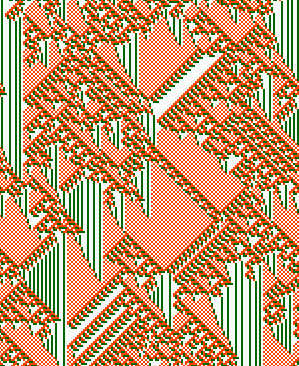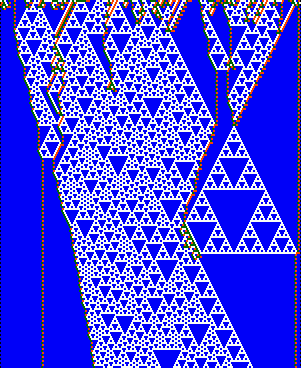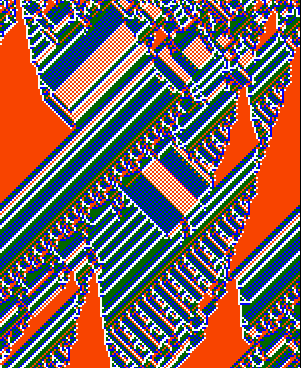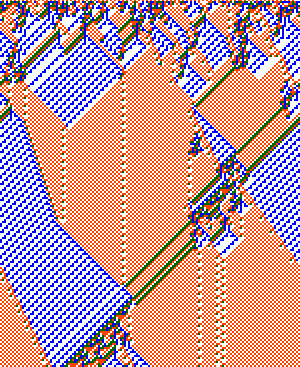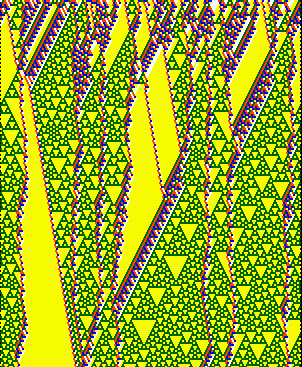v3k3 v4k2 v4k3 v4k3 v5k2 Examples of multi-value complex rules found by the automatic classification method, and included in multi-value DDLab. Note that the k2 examplea have been "un-slanted" (see above). n=150, 183 timesteps from random seeds.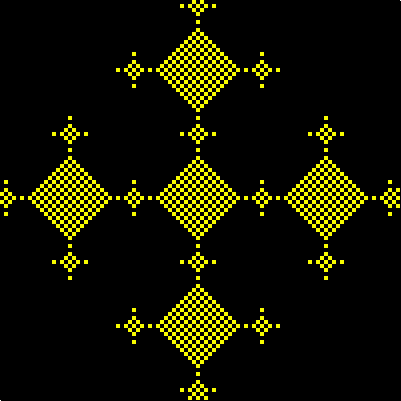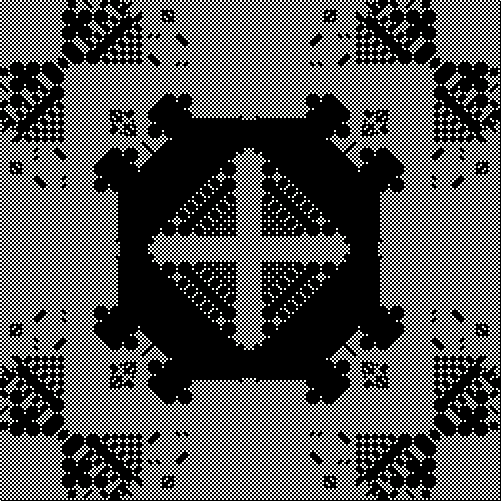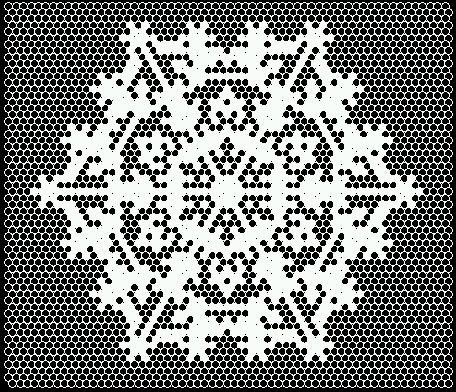v2, k4, 40x40 v2, k4, 250x250, changing the symmetric rule on-the-fly v2, k6, 60x60 shown in hexagonal graph layout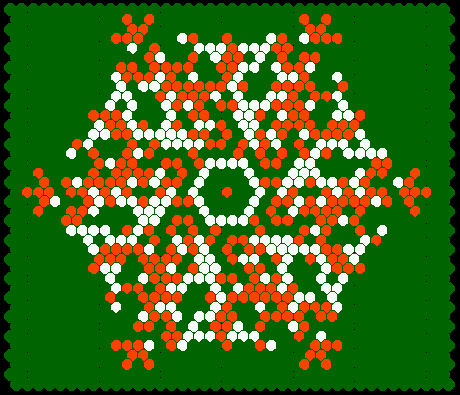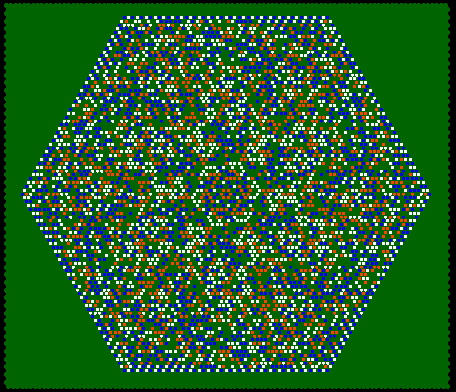v3, k6, 40x40 shown in hexagonal graph layout v4, k7, 100x100 shown in hexagonal graph layout v5, k5, 100x100 Examples of the evolved space patterns of 2d symmetric rules. Starting from a symmetric pattern the symmetry must be conserved.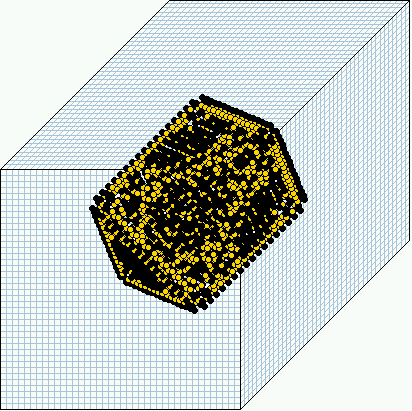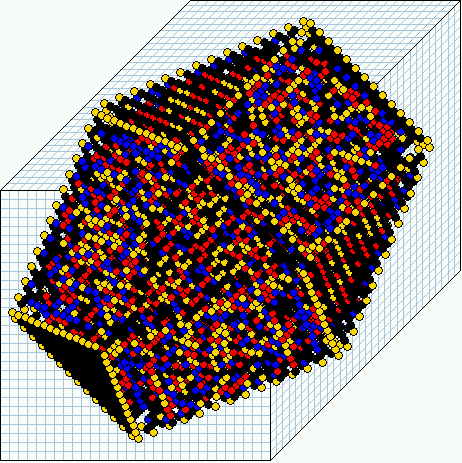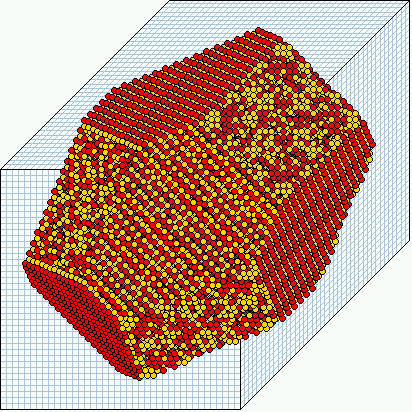v2, k6, 20x20x20 v4, k6, 40x40x40 v5, k6, 30x30x30 v4, k7, 40x40x40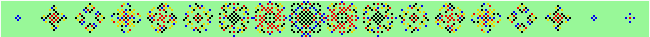v5, k6, 18x18x18, showing successive horizontal slices from left to right. Examples of the evolved space patterns of 3d symmetric rules. Starting from a symmetric pattern the symmetry must be conserved.